# IntroducingHamming Codes

If you’ve used CDs and DVDs before, you’re aware that a small scratch on the disk doesn’t make the disk unplayable; The reader is still able to playback the encoded data almost perfectly, even though there is damage to the data. So how are these readers able to fill in those missing gaps?

One way would be to store 2 duplicates of data and compare each 1 and 0 in the disk to those duplicates to see if they match. If they don’t match, then change the bit at the “damaged” position to the majority value of the duplicates, like this:

``````(spaces are provided for visual clarity)
original:   0111010 0 011001010111 1 0110111010000001010
duplicate:  0111010 1 011001010111 0 0110111010000001010
duplicate:  0111010 0 011001010111 0 0110111010000001010

after corrections:

corrected:  0111010 0 011001010111 0 0110111010000001010
duplicate:  0111010 0 011001010111 0 0110111010000001010
duplicate:  0111010 0 011001010111 0 0110111010000001010
``````

However, this method is extremely inefficient. Although it does point out errors, you end up spending two-thirds of your disk space on duplicate data, when you could be using that space for more useful data. So the question becomes, how do we encode error correction without taking up a large amount of disk space?

One method is using Hamming codes. Let’s say we have 64 bits, each containing either a 1 or a 0. For visual clarity, they will be laid out in an 8 x 8 grid, with each bit being separated like so:

``````bit-0, bit-1, bit-2, bit-3, ... , bit-7,
bit-8, bit-9, bit-10, bit-11, ... , bit-15,
...
``````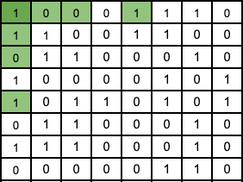There are 6 values (highlighted in light green) that we will use as error-correcting bits, or “parity bits”. You will also notice that the places that these green squares are in are powers of 2 (1, 2, 4, 8, 16, 32, …). These bits tell whether or not the sum of the values within a designated area (shown below) is even or odd, with a 0 in the parity bit if it's even, and a 1 if it’s odd.

The value highlighted in dark green is the total parity of the other 63 bits, following the same rule as the other parity bits.

Using these bits, we can determine if and where there are errors so that we can correct them and end up with the intended encoded data.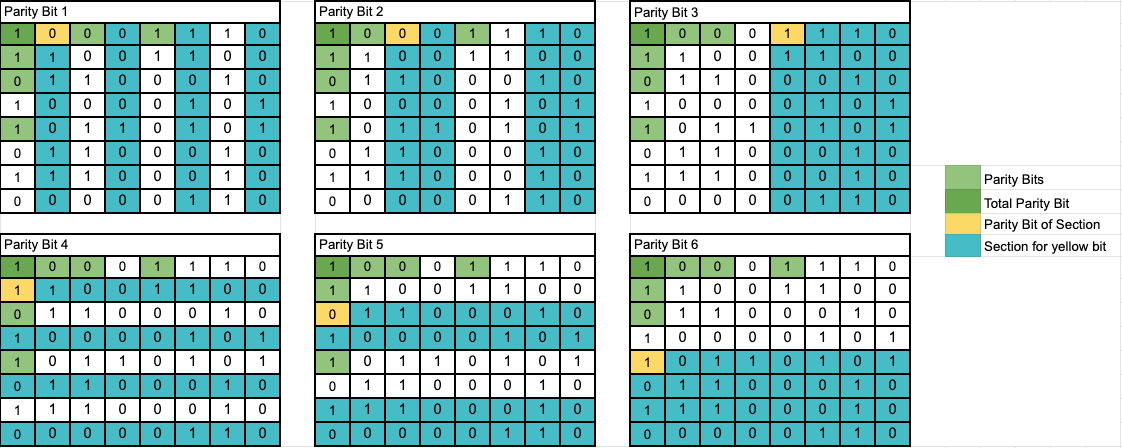Let’s flip a random bit and see how this system of parity bits can identify the mistake.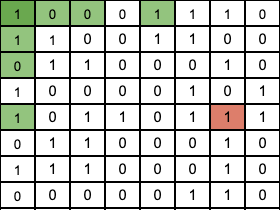Since that value has changed from a 0 to a 1, some of the parity bits will be incorrect, as the sums of their corresponding regions are not what they used to be. So all the computer needs to do is recalculate each parity bit to see if they are correct, and we can narrow the regions down until we locate the incorrect bit.

Parity Bit 1: The computer will add up the 1’s in the blue columns, and see if they are even (parity bit value of 0). Since the sum is an even number, it has passed this check, meaning the error is in the other columns (highlighted in red)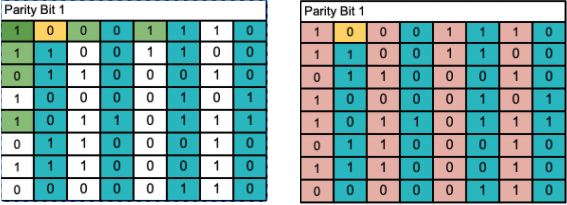Parity Bit 2: Once again, we will add up the 1’s in the blue columns and check the parity of the sum. Here, it did not pass the check, as the sum is not even as the parity bit had stated. We can narrow the error down to two columns by taking the error columns from the first test and combining it with our results from the second.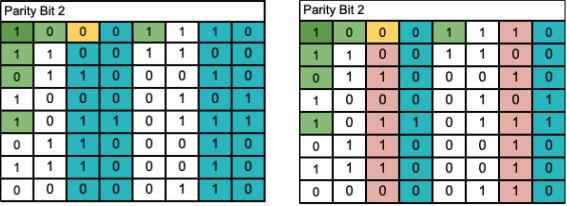Parity Bit 3: Same as the other two checks. This one fails, and now we have narrowed the error down to its column.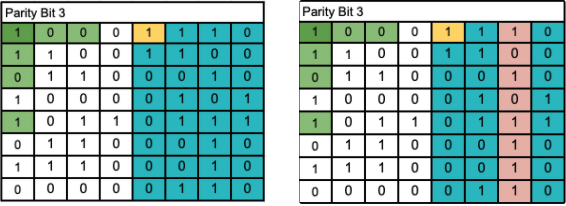Parity Bit 4: Same as the first check, just in rows. This check passes.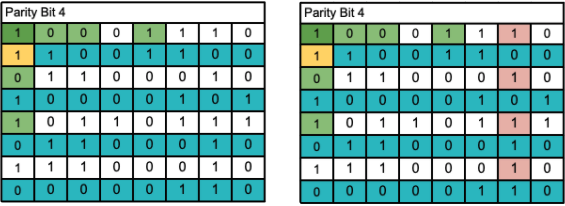Parity Bit 5: This one passes.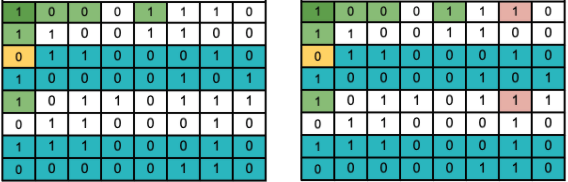Parity Bit 6: This one fails.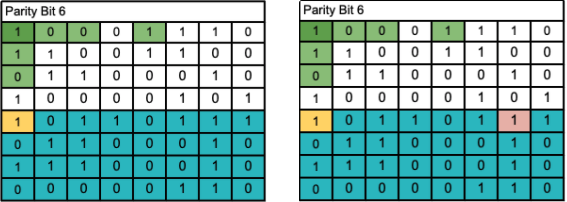With 6 simple checks, we were able to locate the error. From here, the computer can flip this bit around and get the correct encoded data. Of course, this method will work on much bigger sets of data, as long as there are more parity bits to narrow down errors.

This method will also be able to tell if there are multiple errors within each set of bits, but will not be able to correct them. However, for small 1 bit errors, hamming codes are an extremely simple and elegant solution to error correction and is why we can get perfect data, even if there is a small scratch or two.